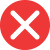22th

Incorrect spelling, explanation: 22th is an incorrect form, because every number which end with 2 has suffix nd in its ordinal form. -th is added to every number except 1, 2 and 3 (their suffixes are respectively -st, -nd and -rd). This is why the correct ordinal form of 22 is 22nd.22nd

Correct spelling, explanation: to create an ordinal number from the cardinal one we usually add suffix -th. There are three exceptions, though: for numbers one, two and three (and all numbers which end with 1,2 and 3). For example 20 as the ordinal number will be 22nd, but 24 will be 24th. This is why the form 22th is incorrect.

Definition of 22nd:
numeral, ordinal number between 21st and 23rd
Her birthday is on 22nd of March.
I started collecting art last year. This painting is my 22nd one.22st

Incorrect spelling22rd

Incorrect spelling